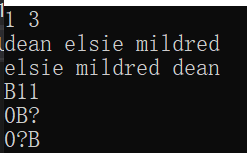# Code works locally but not on the USACO wensite

## Problem Info

Acowdemia II USACO

## My Work

``````//http://www.usaco.org/index.php?page=viewproblem2&cpid=1132
#include<bits/stdc++.h>
using namespace std;

int K, N;
vector<vector<string>>paper;
map<string, int> names;

void testing(vector<vector<string>>a) {//prints out a vector<vector<string>>
for (int i = 0; i < a.size(); i++) {
for (int j = 0; j < a[i].size(); j++) {
cout << a[i][j];
}
cout << endl;
}
}

void update(vector<string>b, int index) { //updates the answer vector based on the paper and index
for (int i = 0; i < index; i++) {
for (int j = index; j < N; j++) {
}
}
}

int main(void) {

cin >> K >> N;

//dictionary for names for quick conversion from name to integer
for (int i = 0; i < N; i++) {
string temp;
cin >> temp;
names.insert({ temp, i });
}
//inputs the names for each paper
for (int i = 0; i < K; i++) {
vector<string>temp;
for (int j = 0; j < N; j++) {
string temps;
cin >> temps;
temp.push_back(temps);
}
paper.push_back(temp);
}
//initialises the answer matrix with all ? except for the diagonal (where values should be B)
for (int i = 0; i < N; i++) {
vector<string>temp;
for (int j = 0; j < N; j++) {
if (i != j) {
temp.push_back("?");
}
else {
temp.push_back("B");
}
}
}

for (int i = 0; i < K; i++) {//for each paper
for (int j = 1; j < N; j++) {//for each name
if (paper[i][j].compare(paper[i][j - 1]) == -1) {
update(paper[i], j);
}
}
}
//The output from my 2019 Visual Studio Code is fine, it passes all test cases i tried from the USACO website
//But when I submit it to the USACO website with C++17 chosen, it prints out the initial answer matrix
//which is the one on line 49

return 0;
}
``````

## What I’ve Tried

I’ve tried to change the datatypes of the answer matrix and the other data associated with it from string to char, but it still didn’t work.

## Question

I don’t understand why my code is failing once I submitted it to the USACO website, but not locally.

## Checklist

• Include a link to the problem
• Format your post (especially code blocks)
• Include what you’ve tried so far

Here are some screenshots from my VS code 2019 IDE and the USACO websiteYou might be accessing memory that’s out of bounds. Are you sure all your vector indices are in the bounds of the vectors?

1 Like

`compare` doesn’t always return a value in [-1,1] (it may be platform-specific behavior). Not sure why you wouldn’t use `<` instead; personally I’ve never used this function.

(Note: This is C++14 instead of C++17 but w/e)

1 Like

Thank you so much. Changing compare to < indeed solved my problem.